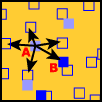# Motion Correspondence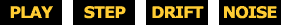## Introduction

When this activity initially loads, you should see a collection of small blue squares arranged in a circular pattern. Most likely, you will perceive the squares to be moving around in a circle. Click the STEP button on the left several times. The motion will stop and you will see that there are actually only two frames in this movie, each with eight squares in alternating positions around the circle.

If you have already completed the activity on Types of Motion, you will probably recognize this as an example of apparent motion. When the movie is played (click the PLAY button to start it again), instead of perceiving 16 squares flashing on and off, you instead perceive eight squares moving around in a circle. But unlike the demonstration in the previous activity, the direction of motion here is ambiguous. You should sometimes see the squares moving clockwise and other times moving counterclockwise.

The ambiguity arises because each square in frame 1 could potentially correspond to one of two different squares in frame 2: one square immediately clockwise or counterclockwise from the reference square in frame 1. Given that there is more than one possible matching square, which one should your visual system choose? This correspondence “problem” is similar to the one we faced when studying binocular vision (Chapter 6). There, the issue was which points on the left retina corresponded with which points on the right retina. Here, the issue is which points in the image we see at time 1 correspond to which points in the image at time 2? As noted in the chapter on binocular vision, correspondence is only a “problem” for the researchers studying motion perception. The visual system itself is remarkably adept at solving the problem in everyday life.

### Instructions

This activity illustrates several different aspects of motion correspondence.

Click on the buttons at left to do the following:

• PLAY starts the movie playing (i.e., the two frames of the movie will start alternating on and off).
• STEP stops the movie and allows you to alternate manually back and forth between the two frames.
• DRIFT starts the movie (whether playing or stopped) moving across the screen in random directions (when it reaches the edge of the screen it will change direction).
• NOISE adds 130 randomly-placed static squares behind the movie.

Click the links at left to read about the correspondence issues you will encounter with different combinations of drift and noise settings.

## Movie Playing, Not Drifting, No Noise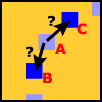The correspondence problem occurs because each square in frame 1 could correspond to either of two squares in frame 2. This is illustrated in the figure above (in this figure the light blue squares are visible in frame 1 and the dark blue squares are visible in frame 2). Matching square A in frame 1 with square B in frame 2 would lead to the perception of counterclockwise motion. On the other hand, if square A is taken to correspond to square C, clockwise motion would be perceived.

Researchers have discovered a number of principles that the visual system uses to interpret ambiguous motion stimuli such as this. For the display at left, we have already seen that the direction of motion for any particular square is ambiguous, but note that you generally perceive all the squares as moving in the same direction. This fact in itself shows one principle at work: once the direction is established for one square (really, a pair of squares), the visual system matches all the other squares so as to make the global direction of motion consistent.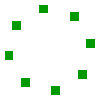The movie above demonstrates another principle: Match each object in frame 1 with the closest object in frame 2. In the movie at left, squares B and C in frame 2 are equidistant from square A in frame 1, so this principle cannot be applied. The frames in the movie above are identical to those in the main display, except that the squares in frame 1 have been rotated a bit clockwise and the squares in frame 2 rotated a bit counterclockwise. Now square A in frame 1 is closer to square C than to square B in frame 2. As a result, you are probably more likely to perceive the squares as oscillating back and forth rather than moving around the circle in one direction or another.

Now stop the movie at left (click the STEP button) and click the DRIFT button to illustrate another correspondence “problem.”

Click on "Movie Stopped, Drifting, No Noise" for a description.

## Movie Stopped, Drifting, No Noise

Here the circle of squares is “drifting” around the screen in random directions and now we face a new correspondence problem. Although the two frames of our movie are not alternating back and forth, all the squares in the frame we see are shifting position every 150 milliseconds. Therefore, any square at time 1 might correspond to any square at time 2 (after the squares have shifted position).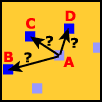The figure above diagrams this situation, showing the squares moving leftward and slightly upward from time 1 (light blue squares) to time 2 (dark blue squares). Square A at time 1 could go with square B, C, or D at time 2. How should we solve the correspondence problem in this case?

One strategy would be to consider each individual square separately and apply the shortest distance criterion, matching each square at time 1 with the closest square at time 2. This would work for some squares, but in the section of the circle diagrammed above, this rule would lead us to mistakenly match square A with square D.

To cope with situations similar to these, the visual system instead calculates global motion across large portions of the retina, rather than just matching up individual points. These global motion detectors appear to reside in the medial temporal region of the cortex (known as area MT).

Now click the PLAY button to start alternating the frames of the movie as the circle of squares drifts around. Do you still perceive the squares as rotating around the circle?

Click on "Movie Playing, Drifting, No Noise" to move on to the next part of this module.

## Movie Playing, Drifting, No Noise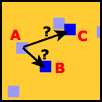Here the circle of squares is both drifting and alternating frames. Thus, two correspondence problems are combined: We must match squares both across frames and across time, as illustrated above (in which frame 1 at time 1 is shown in light blue and frame 2 at time 2 in dark blue).

Rather remarkably, your visual system perceives the squares as both rotating around the circle and drifting at the same time. In fact, the perception of rotation may be even stronger in this case than when the squares were not drifting. Note, however, that with drifting turned on, the direction of rotation is less ambiguous. For example, if the squares are drifting to the right, they will usually appear to rotate counterclockwise (click here to push the squares to the far left of the screen and start them moving directly rightward).

The ambiguity of rotation direction is resolved in this case because of the shortest distance constraint. As shown in the diagram above, square A at time 1 could be matched with either square B or C at time 2. When the squares are not drifting, the distance to B and C is equivalent, but with drifting turned on, one of the two options becomes closer than the other. This is true, and consistent, across all the squares in the movie. Therefore, the visual system usually settles on the rotation direction that leads to the shortest distance between squares at time 1 and at time 2.

Now click the NOISE button to see what happens when we add extra squares in the background.

Click "Adding Noise" for an explanation of what adding noise does to the display.